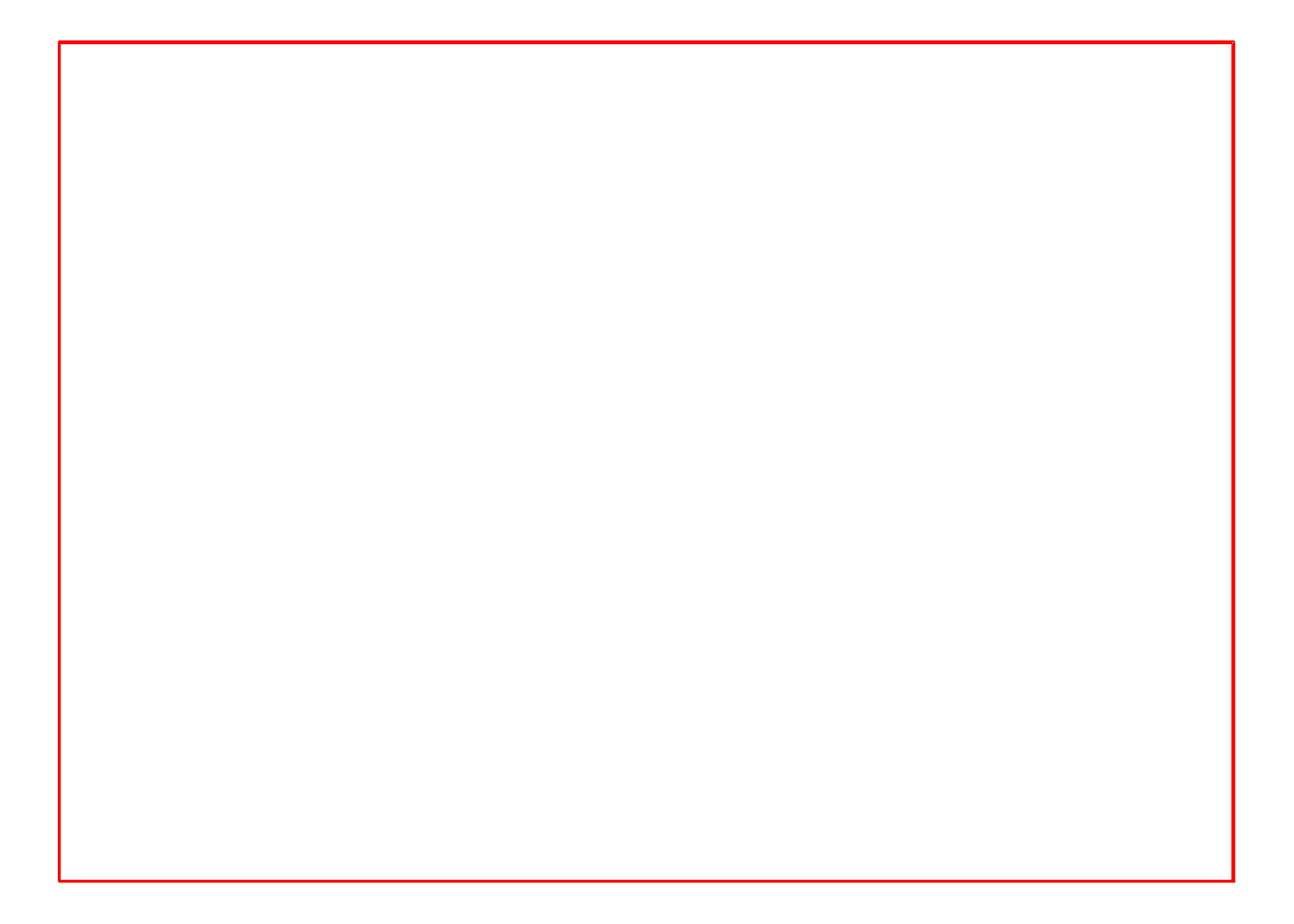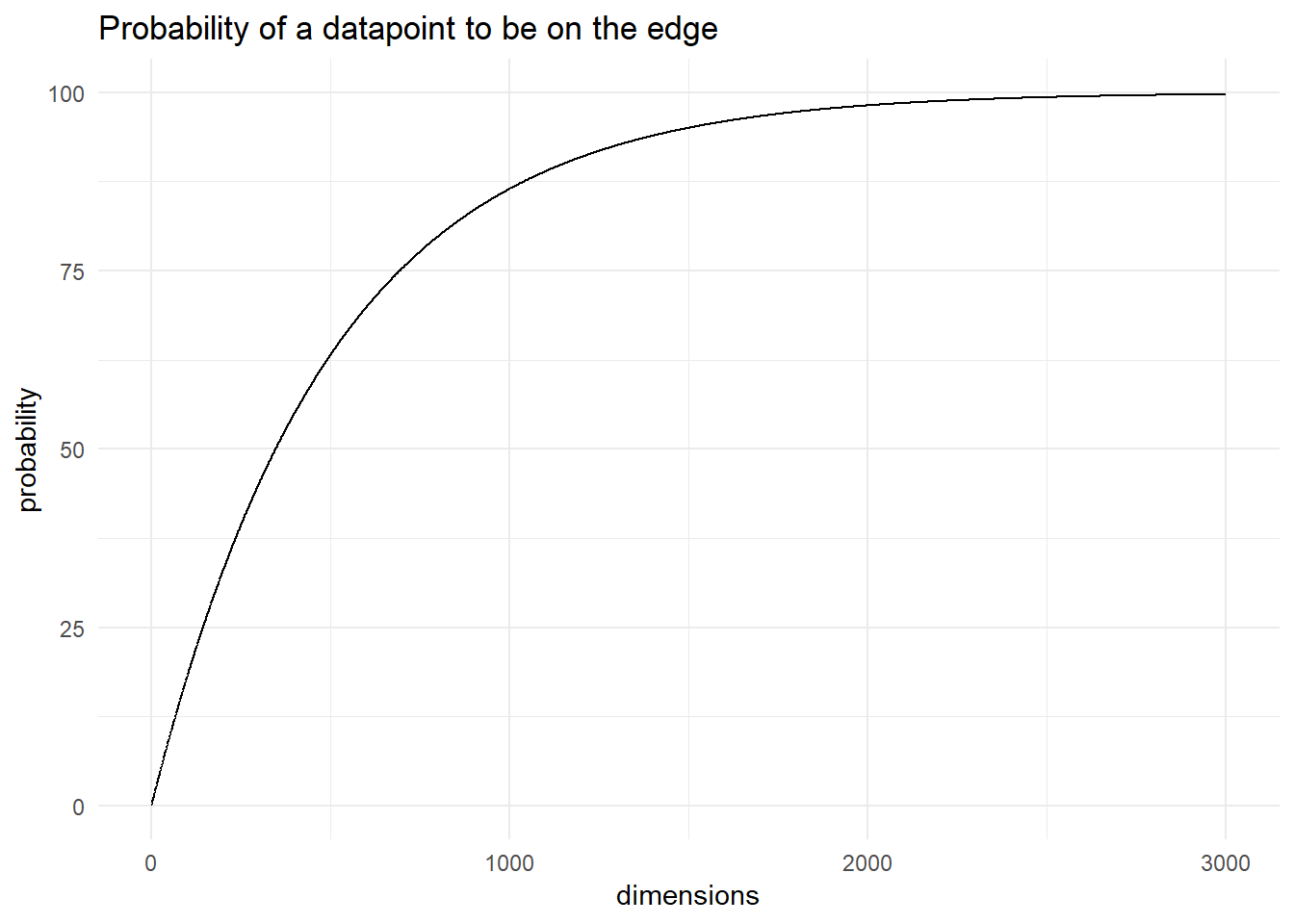# Curse of dimensionality

20/03/2020

## Multi dimension intuition

Because we are all used to two or three dimensions, we are not used to multidimensional thinking. In this blog, I want to show the curse of dimensionality using an example.

Take a classification problem, for example. In a classification problem, the goal is to define a distinct boundary between the classes. The probability of a data point to be of a particular class increases as it is farther away from the decision boundary.

Pick a random point from a line of unit length. The probability of the point being in the edges (defined by <0.001 from the border) is
$p = \frac{2\times 0.001}{1} = 0.002 = 2\%$Similarly, in a two-dimensional space, consider a unit square. The probability of a point being in the edges is:
$p = 1- \frac{area\,of\,square\,of\,0.998\,side}{area\,of\,unit\,square} = 1 - \frac{0.998*0.998}{1*1} = 0.003996 = 4\%$In a three dimensional space, in a unit cube, the probability of a point being in the edges is:
$p = 1- \frac{area\,of\,cube\,of\,0.998\,length}{area\,of\,unit\,cube}= 1 - \frac{0.998\times 0.998\times 0.998}{1\times1\times1} = 0.005988008 = 5\%$

similarly, in an n-dimensional space, the probability would be: $p = 1 - 0.998^n$ As ‘n’ increases, the probability of a data point is on the edges (defined by <0.001 from the border) is:For just 50 dimensions, almost 10% of the data is on the edges. At 3000 dimensions, almost 99.75% of the data is on the edges.

In problems with a large number of features, a very high proportion of the data would be at the edges. This is called the curse of dimensionality and is the reason why feature engineering is essential.